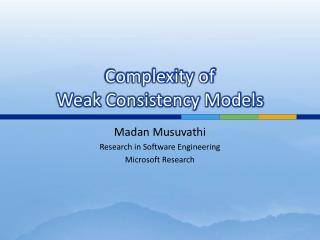# Complexity of Weak Consistency Models - PowerPoint PPT PresentationDownload PresentationComplexity of Weak Consistency Models

Complexity of Weak Consistency ModelsDownload Presentation## Complexity of Weak Consistency Models

- - - - - - - - - - - - - - - - - - - - - - - - - - - E N D - - - - - - - - - - - - - - - - - - - - - - - - - - -
##### Presentation Transcript

1. Complexity of Weak Consistency Models Madan Musuvathi Research in Software Engineering Microsoft Research

2. Weak Consistency Models • Hard to program • Hard to test • Hard to debug • General consensus: trade consistency for • Availability • Performance • Partition-tolerance • …

3. Quantifying the Cost of Weak Consistency • How hard are weak consistency models to program/test/debug? • Study the hardness of associated verification problems • Assumption: • Programming models that are hard for computers to reason are also hard for humans

4. Memory Consistency Models • A memory consistency model is an abstraction of the memory subsystem • Defines when and in what order memory operations from one thread are visible to others • Sequential consistency is what programmers want, but is expensive

5. Quiz: Can this assert fail ? Answer: depends on the memory model ! Init: x = 0; y = 0 x = 1; t = y; y = 1; u = x; assert( ! (t == 0&& u == 0) )

6. Sequential Consistency Can the assert fail? No Init: x = 0; y = 0 x = 1; t = y; y = 1; u = x; assert( ! (t == 0 && u == 0) ) SC

7. x86 Memory Model Can the assert fail? Yes Init: x = 0; y = 0 x = 1; t = y; y = 1; u = x; assert( ! (t == 0 && u == 0) ) x86

8. Memory Model Verification Problem • Safety verification • Does this system reach a bad state • Liveness verification • Does this system repeatedly reach a good state Finite State Machine Finite State Machine Finite State Machine … Memory Model Memory (finite)

9. Complexity of Memory Model Verification [POPL ‘10] • Formalizes the intuition that SC is easier to program than relaxed memory models

10. Shared Memory vs Message Passing Decidable Undecidable Finite State Machine Finite State Machine Finite State Machine … Shared Memory Finite State Machine Finite State Machine Finite State Machine … Message Passing Network

11. Conclusion • Weak consistency comes at a cost of programmability/testability/debuggability • Studying the associated verification problem can quantify this cost • Future: Formalize weak consistency models for the cloud